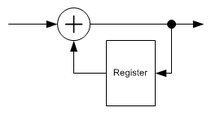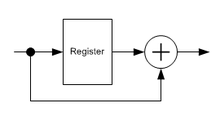# Differential coding

﻿
Differential coding

In digital communications, differential coding is a technique used to provide unambiguous signal reception when using some types of modulation. It makes data to be transmitted to depend not only on the current bit (or symbol), but also on the previous one.

The common types of modulation that require differential coding include phase shift keying and quadrature amplitude modulation.

## Purposes of differential coding

To demodulate BPSK one needs to make a local oscillator synchronous with the remote one. This is accomplished by a carrier recovery circuit. However, a carrier can be recovered in different ways, depending upon a valid phases count (2 for BPSK).

For this coding, if a carrier is recovered incorrectly, the received data are inverted.

Assuming that xi is a bit intended for transmission, and yi is a bit actually transmitted (differentially encoded), if$y_i=y_{i-1} \oplus x_i, \qquad (1)$

is transmitted, then on the decoding side$x_i=y_i \oplus y_{i-1}. \qquad (2)$

can be reconstructed, where$\oplus{}$ indicates binary or modulo-2 addition.

Now xi depends only on a difference between yi and yi − 1 and not on their values. So, whether the data stream is inverted or not, the decoded data will always be correct.

When data is transmitted over twisted-pair wires, it is easy to accidentally insert an extra half-twist in the cable between the transmitter and the receiver. When this happens, the received data are inverted. There are several different line codes designed to be polarity insensitive -- whether the data stream is inverted or not, the decoded data will always be correct. The line codes with this property include differential Manchester encoding, bipolar encoding, NRZI, biphase mark code, coded mark inversion, and MLT-3 encoding.

## Conventional differential codingA differential encoderA differential decoder

A method illustrated above can deal with a data stream inversion (it is called 180° ambiguity). Sometimes it is enough (e.g. if BPSK is used or if other ambiguities are detected by other circuits, such as a Viterbi decoder or a frame synchronizer) and sometimes it isn't.

Generally speaking, a differential coding applies to symbols (these are not necessary the same symbols as used in the modulator). To resolve 180° ambiguity only, bits are used as these symbols. When dealing with 90° ambiguity, pairs of bits are used, and triplets of bits are used to resolve 45° ambiguity (e.g. in 8PSK).

A differential encoder provides the (1) operation, a differential decoder - the (2) operation.

Both differential encoder and differential decoder are discrete linear time-invariant systems. The former is recursive and IIR, the latter is non-recursive and thus FIR. They can be analyzed as digital filters.

A differential encoder is similar to an analog integrator. It has an impulse response$h(k)=\begin{cases} 1, & \mbox{if } k \ge 0 \\ 0, & \mbox{if } k < 0 \end{cases}$

and a transfer function$\ H(z)=\frac{1}{1-z^{-1}}.$

A differential decoder is thus similar to an analog differentiator, its impulse response being$h(k)=\begin{cases} 1, & \mbox{if } k=0 \\ -1, & \mbox{if } k=1 \\ 0, & \mbox {otherwise} \end{cases}$

and its transfer function$\ H(z)=1-z^{-1}.$

Note that in binary (modulo-2) arithmetic, addition and subtraction (and positive and negative numbers) are equivalent.

## Generalized differential coding

Using the relation$y_{i-1} \oplus x_i = y_i$ is not the only way of carrying out differential encoding. More generally, it can be any function u = F(y,x) provided that an equation u0 = F(y0,x) has one and only one solution for any y0 and u0.

## Applications

Differential coding is widely used in satellite and radio relay communications together with PSK and QAM modulations.

## Drawbacks

Differential coding has one significant drawback: it leads to error multiplication. That is, if one symbol such as yi was received incorrectly, two incorrect symbols xi and xi + 1 would be at the differential decoder's output, see:$x_i=y_i \oplus y_{i-1}$ and$x_{i+1}=y_{i+1} \oplus y_i$. This approximately doubles the BER at signal-to-noise ratios for which errors rarely occur in consecutive symbols.

## Other techniques to resolve a phase ambiguity

Differential coding is not the only way to deal with a phase ambiguity. The other popular technique is to use sync-words for this purpose. That is, if a frame synchronizer detects repeated inverted sync-words, it inverts the whole stream. This method is used in DVB-S.

• INTELSAT Earth Station Standard IESS-308
• DVB framing structure, channel coding and modulation for 11/12 GHz satellite services (EN 300 421)

Wikimedia Foundation. 2010.

### Look at other dictionaries:

• Differential Manchester encoding — Differential Manchester encoding, also called biphase mark code (BMC) or FM1, is a line code in which data and clock signals are combined to form a single 2 level self synchronizing data stream. It is a differential encoding, using the presence… …   Wikipedia

• Differential execution — refers to a method of executing a computer subroutine (See control flow) in such a way that differences from prior executions can be detected and acted upon. If the subroutine is one that walks through a data structure, differential execution can …   Wikipedia

• Golomb coding — is a data compression scheme invented by Solomon W. Golomb in the 1960s. The scheme is based on entropy encoding and is optimal (in the sense of Shannon s source coding theorem) for alphabets following a geometric distribution, making it highly… …   Wikipedia

• Video Coding Experts Group — The Video Coding Experts Group or VCEG is the informal name of Question 6 (Video coding) of Working Party 3 (Media coding) of Study Group 16 (Multimedia terminals, systems and applications) of the ITU T. It is responsible for standardization of… …   Wikipedia

• Long non-coding RNA — Long noncoding RNAs (long ncRNAs) are generally considered (somewhat arbitrarily) as non protein coding transcripts longer than 200 nucleotides. This limit is due to practical considerations including the separation of RNAs in common experimental …   Wikipedia

• Shannon's source coding theorem — In information theory, Shannon s source coding theorem (or noiseless coding theorem) establishes the limits to possible data compression, and the operational meaning of the Shannon entropy.The source coding theorem shows that (in the limit, as… …   Wikipedia

• Adaptive Differential Pulse Code Modulation — (ADPCM), auch Delta Pulse Code Modulation genannt, ist eine komprimierende Kodierung für ein Signal welche ähnlich wie Differential Pulse Code Modulation (DPCM) auf Differenzwerten basiert und zusätzlich die Skalierung der Quantisierungsstufen in …   Deutsch Wikipedia

• Adaptive Coding and Modulation — (ACM) ist ein Verfahren der Datenübertragung, bei der der Empfänger eine Rückmeldung über die Qualität der empfangenen Daten gibt und darüber die Datenkodierung und Datenmodulation des Datenübertragungskanals anpasst. Beispielsweise wird ACM bei… …   Deutsch Wikipedia

• Transition Minimized Differential Signaling — (TMDS) is a technology for transmitting high speed serial data and is used by the DVI and HDMI video interfaces, as well as other digital communication interfaces. It replaces RAMDAC, used in analog VGA video interface.The transmitter… …   Wikipedia

• Phase-shift keying — Passband modulation v · d · e Analog modulation AM · …   Wikipedia